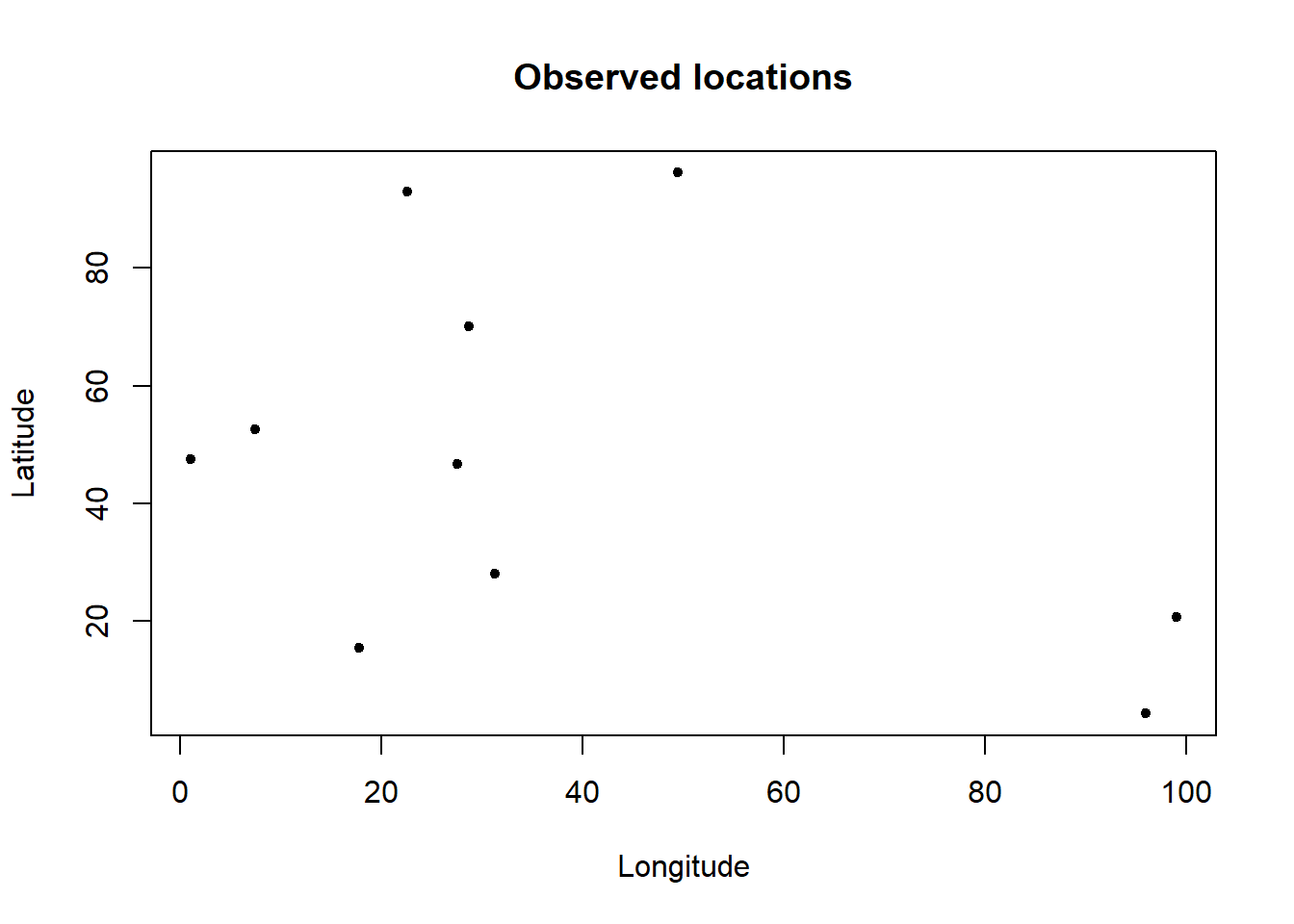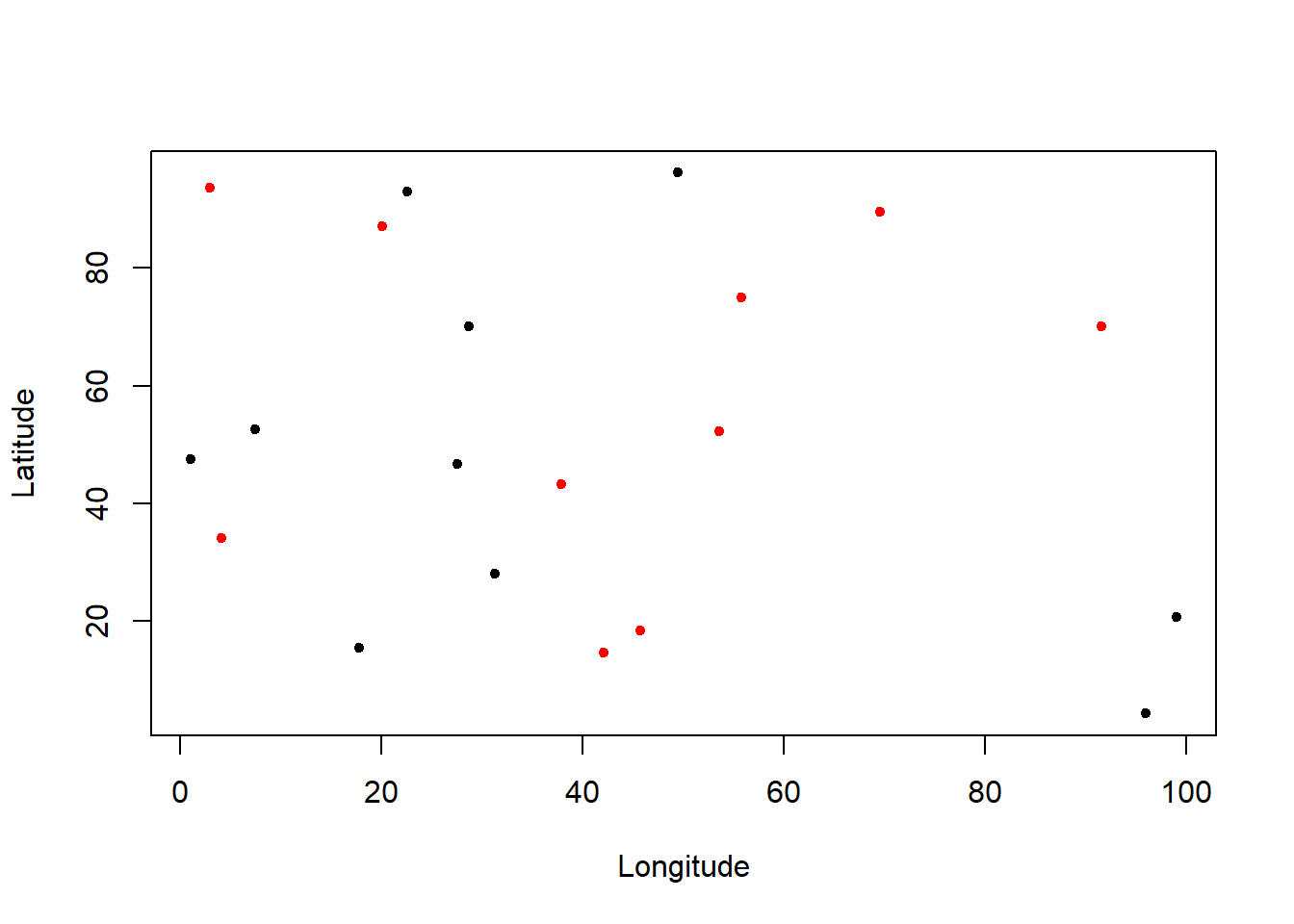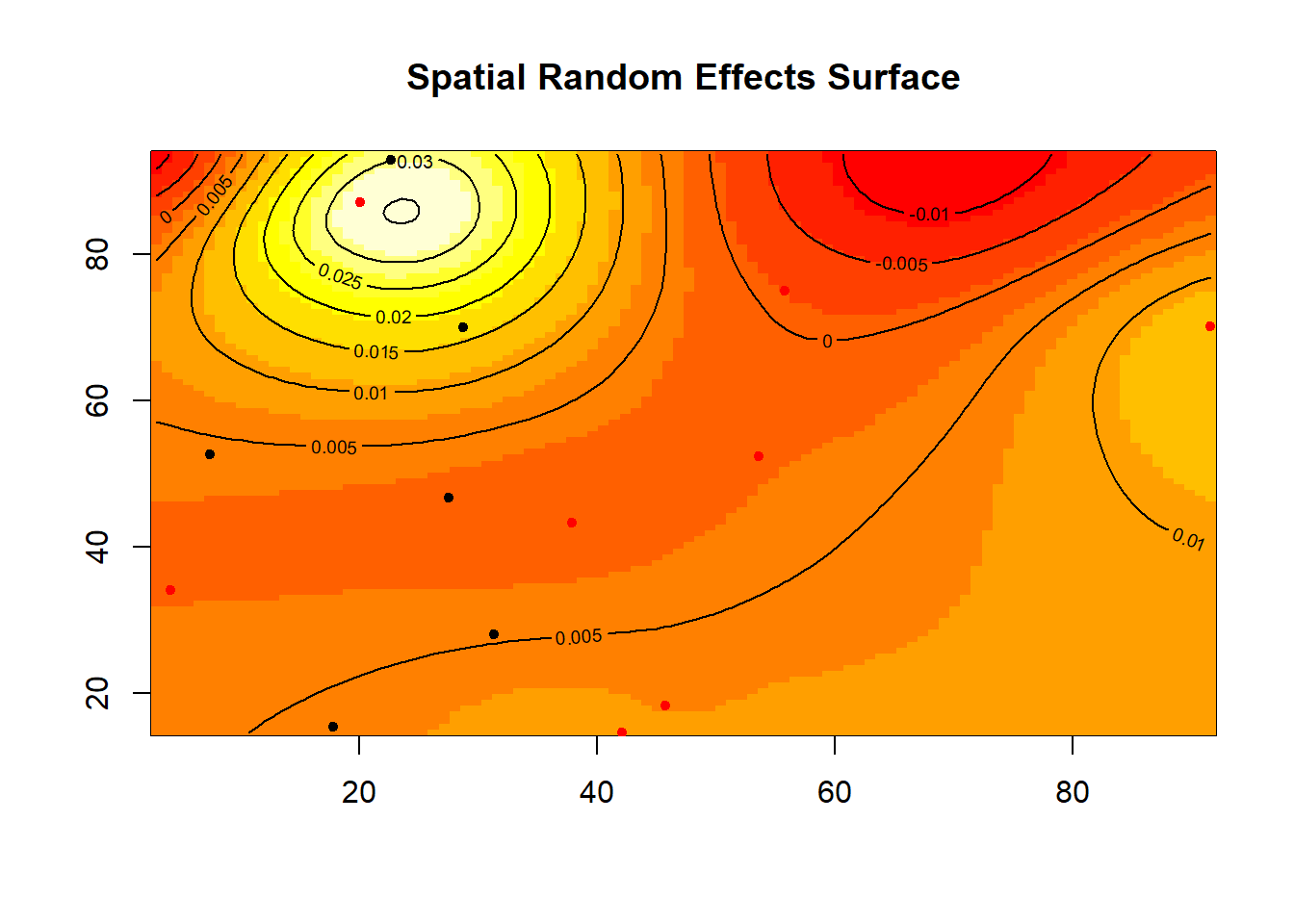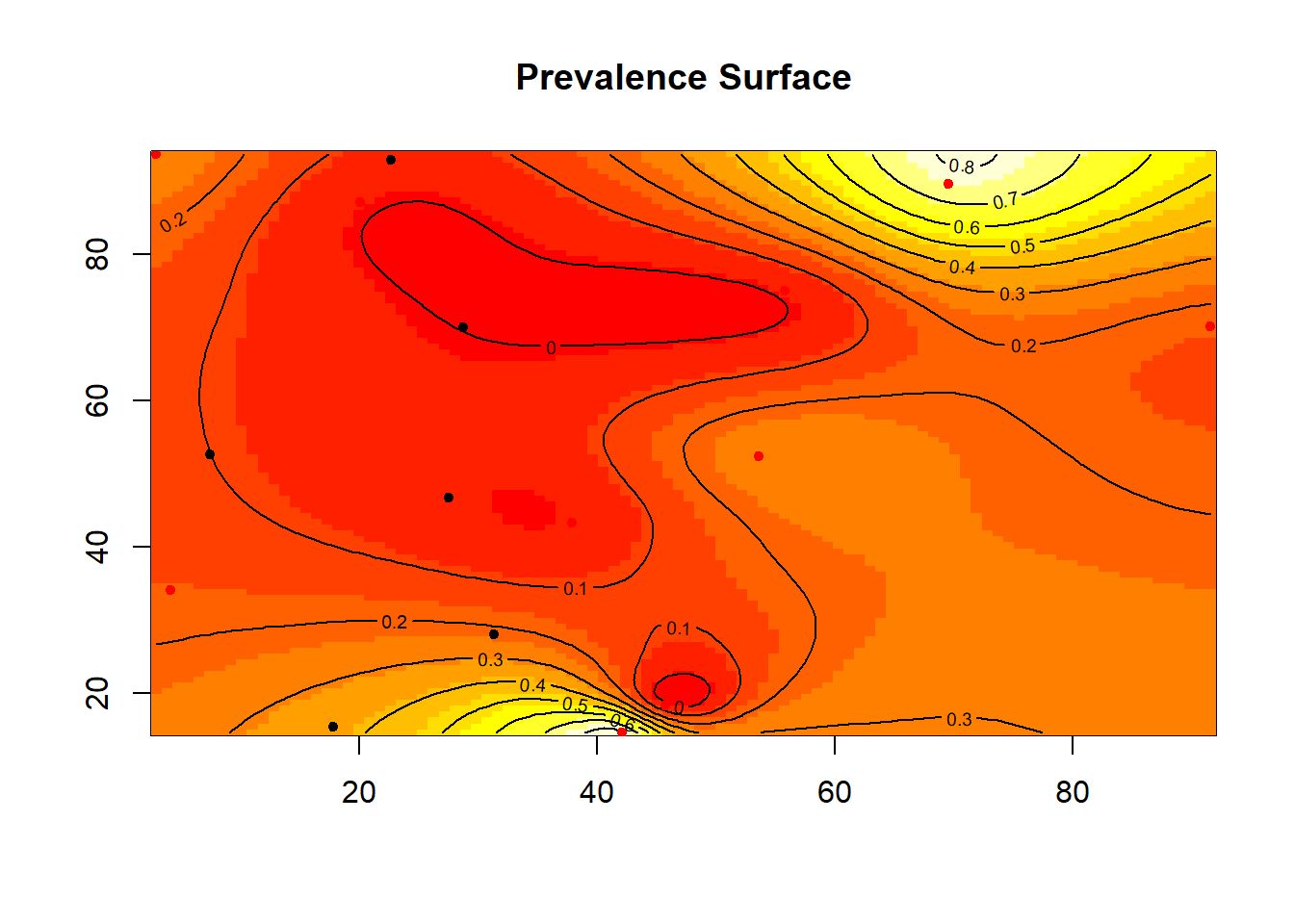## Introduction

One of the most widespread uses of Demographic and Health Survey (DHS) data is to estimate the prevalence of various diseases or exposures across a particpating country. The motivation for doing so is often to help better inform public health decision-making by making prevalence estimates more localized. That is, instead of working with prevalence estimates at an administrative level, such as a province, kriging produces a smooth surface, where a prevalence estimate varies continuously over space. Since this is a widely used procedure, and becomming more so now that the Global Burden of Disease project is moving towards more localized estimates, this document briefly introduces kriging, and demonstrates it using two different R libraries.

## The Basics

The first thing to understand about kriging is that it is simply an extension of regression models. In this sense, the technique may be a familiar one to most public health practitioners, who, even if not trained in statistics, have no doubt been exposed to regression analysis in one way or another. Additionally, kriging is part of a wider class of models known as multilevel models. To begin to unpack this, we write the regression model for a single location as follows:

$y_i = \mathbf{x_i}^T\mathbf{\beta} + \theta_i + \epsilon_i$

where $$y_i$$ is a response variable, such as the prevalence of a disease in a location, $$\mathbf{x_i}^T$$ is a p-dimensional row vector of covariates that are presumed to be associated with the response variable, $$\mathbf{\beta}$$ is a $$p \times 1$$ vector of regression coefficients linking the covariates to the response, while $$\epsilon_i$$ is an uncorrelated, white noise error. The $$\theta_i$$ term is also an error term, but is spatially correlated. These $$\theta$$’s are generally referred to as spatial random effects. Epidemiologically, we can interpret them as unmeasured confounding that exhibits spatial structure. This structure, then, is what allows us to make predictions. It does so by modeling residual spatial variability. The question then becomes: How does one model it? Within a multilevel modeling framework, this is done by placing a Gaussian Process prior on the vector $$\mathbf{\theta}$$. The Gaussian process prior is simply a multivariate normal distribution that has dimension equal to the number of points in the dataset, with the spatial correlation induced in the covariance matrix of the prior. To understand this prior a bit better, we can write this prior as follows:

$\mathbf{\theta}|\sigma^2,\phi \sim \text{N}(\mathbf{0},\sigma^2\mathbf{\Sigma}(\phi))$

Here, two more parameters have been introduced, $$\sigma^2$$ and $$\phi$$. The former represents the variance of the spatial process (i.e. the Gaussian process), while $$\phi$$ represents the range of the process, or, in other words, the rate of decay in spatial correlation. Finally, $$\mathbf{\Sigma}()$$ represents a correlation function, for example an exponential correlation function.

$\mathbf{\Sigma}(\phi) = \text{exp}(-\phi\mathbf{D})$

where $$\mathbf{D}$$ is a matrix of pairwise distances between points, and the correlation decays exponentially as the distance between points increases, and does so at a rate governed by $$\phi$$.

With these basics in hand, we now turn to fitting the model. There are a number of options available to do so within the R software environment. We focus on spBayes, as it offers a flexible class of modeling options for point-reference, spatial and spatio-temporal data.

## Fitting a spatial logistic regression model

We begin by loading spBayes and the MBA libraries. As noted, the former will be used for model fitting, while the latter will be used for mapping.

library(MBA)
library(spBayes)
## Loading required package: coda
## Loading required package: magic
## Loading required package: abind
## Loading required package: Formula

Note that by loading these libraries, a number of other packages were also loaded. These are typically necessary for managing spatial data within R (the sp libraries loaded by MBA), or doing Bayesian analysis (e.g. the code library loaded by spBayes).

With these two libraries loaded, we can now simulate some spatial data. As an aside, simulation is a useful tool for ensuring that you implement software correctly. That is, if you want to know whether or not you are using software correctly, it is best to simulate data first, where you control the truth, and see if your use of the software can recover it. This is why we show the process for doing so here. We begin by setting the seed and defining a function ‘theta’ that will generate the simulated random effects

set.seed(467)
# Define function to draw from multivariate normal distribution
theta <- function(n, mu=0, V = matrix(1)){
p <- length(mu)
if(any(is.na(match(dim(V),p))))
stop("Dimension problem!")
D <- chol(V)
t(matrix(rnorm(n*p), ncol=p) %*% D + rep(mu,rep(n,p)))
}

Next, we begin to simulate some data. The following code chunk generates and plots 10 locations distributed across a unit square of dimension $$100 \times 100$$, which could represent, for example, latitude and longitude:

coords <- as.matrix(cbind(runif(10,0,100),runif(10,0,100)))
plot(coords,pch=20,xlab="Longitude",ylab="Latitude",main="Observed locations")We next begin to simulate the rest of the data, including the random effects $$\mathbf{\theta}$$, a covariate vector $$\mathbf{x}$$, the regression coefficients $$\mathbf{\beta}$$, and the response vector $$\mathbf{y}$$

# Number of observations
n <- nrow(coords)

# True value of rate parameter phi
phi <- 3/10

# True value of spatial variance sigma^2
sigma.sq <- 1

# True Spatial covariance matrix
W <- sigma.sq*exp(-phi*as.matrix(dist(coords)))

# True random effects
w <- theta(1,rep(0,n),W)

# Covariate
x <- as.matrix(cbind(rep(1,n),rnorm(n,0,1)))

# True beta (intercept and slope)
beta <- c(-1,1)

# probability that y=1
p <- exp(x%*%beta + w)/(1 + exp(x%*%beta + w))

# Weights argument passed to spBayes (i.e. the number of Bernoulli trials conducted at each location--1 in our case)
weights <- rep(1,n)

# Now simulate the response
y <- rbinom(n,size=weights,prob=p)

Now that we have all the data simulated, we proceed to fit the model, beginning with some initial values and tuning parameters to help make the MCMC algorithm converge more quickly.

# Specify initial values and tuning parameters
fit <- glm(y~x-1,weights=weights,family="binomial")
initial.beta <- coefficients(fit)
tuning.beta <- t(chol(vcov(fit)))

# spBayes uses adaptive MCMC to obtain posterior estimates, so specify number of batches and number of posterior samples per batch

n.batches <- 100
length.batch <- 200

# Total number of posterior samples is n.batch * length.batch
n.samples <- n.batches*length.batch

We are now ready to fit the model, which will run for 20,000 iterations. Typically longer runs are necessary (such as 100,000 iterations), but our interest here is showing the code to fit the model.

m1 <- spGLM(y~x-1, family="binomial", coords=coords, weights=weights,
starting=list("beta"=initial.beta, "phi"=0.06,"sigma.sq"=1, "w"=0),
tuning=list("beta"=tuning.beta, "phi"=0.5, "sigma.sq"=0.5, "w"=0.5),
priors=list("beta.norm"=list(rep(0,2),diag(10,2)), "phi.Unif"=c(3/100, 3/1), "sigma.sq.IG"=c(2, 1)),
amcmc=list("n.batch"=n.batches, "batch.length"=length.batch, "accept.rate"=0.43),
cov.model="exponential", verbose=TRUE, n.report=10)
## ----------------------------------------
##  General model description
## ----------------------------------------
## Model fit with 10 observations.
##
## Number of covariates 2 (including intercept if specified).
##
## Using the exponential spatial correlation model.
##
## Number of MCMC samples 20000.
##
## Priors and hyperpriors:
##  beta flat.
##
##  sigma.sq IG hyperpriors shape=2.00000 and scale=1.00000
##
##  phi Unif hyperpriors a=0.03000 and b=3.00000
##
## Adaptive Metropolis with target acceptance rate: 43.0
## -------------------------------------------------
##      Sampling
## -------------------------------------------------
## Batch: 10 of 100, 10.00%
##  parameter   acceptance  tuning
##  beta     53.5        2.13350
##  beta     70.0        1.08559
##  sigma.sq    66.5        0.55814
##  phi     89.0        0.55814
## -------------------------------------------------
## Batch: 20 of 100, 20.00%
##  parameter   acceptance  tuning
##  beta     46.0        2.35788
##  beta     68.0        1.19976
##  sigma.sq    60.5        0.61684
##  phi     89.5        0.61684
## -------------------------------------------------
## Batch: 30 of 100, 30.00%
##  parameter   acceptance  tuning
##  beta     49.0        2.40552
##  beta     70.0        1.32594
##  sigma.sq    48.0        0.68171
##  phi     88.5        0.68171
## -------------------------------------------------
## Batch: 40 of 100, 40.00%
##  parameter   acceptance  tuning
##  beta     48.0        2.50369
##  beta     68.5        1.46539
##  sigma.sq    53.0        0.75341
##  phi     85.5        0.75341
## -------------------------------------------------
## Batch: 50 of 100, 50.00%
##  parameter   acceptance  tuning
##  beta     46.5        2.45411
##  beta     50.0        1.61951
##  sigma.sq    46.5        0.81616
##  phi     80.5        0.83265
## -------------------------------------------------
## Batch: 60 of 100, 60.00%
##  parameter   acceptance  tuning
##  beta     43.0        2.45411
##  beta     61.5        1.78983
##  sigma.sq    42.5        0.84947
##  phi     78.0        0.92022
## -------------------------------------------------
## Batch: 70 of 100, 70.00%
##  parameter   acceptance  tuning
##  beta     49.5        2.50369
##  beta     56.5        1.97807
##  sigma.sq    44.0        0.90199
##  phi     77.0        1.01700
## -------------------------------------------------
## Batch: 80 of 100, 80.00%
##  parameter   acceptance  tuning
##  beta     51.5        2.45411
##  beta     55.0        2.18611
##  sigma.sq    39.5        0.90199
##  phi     82.5        1.12395
## -------------------------------------------------
## Batch: 90 of 100, 90.00%
##  parameter   acceptance  tuning
##  beta     42.0        2.31119
##  beta     48.0        2.36818
##  sigma.sq    42.5        0.92022
##  phi     78.0        1.24216
## -------------------------------------------------
## Sampled: 20000 of 20000, 100.00%
## -------------------------------------------------

Now that the model has been run, we can now turn to the kriging step, namely, predicting the spatial random effects to new locations. This is covered in the next section.

## The Kriging Step

The first thing to note about kriging is that it is a post-model fitting process. This idea is made clear by the fact that kriging is fundamentally about prediction. Thus, as in any regression scenario, prediction proceeds only after initially fitting the model to data. Indeed, this is a necessary step because, in order to make a prediction about a process, you need to have an understanding of the parmeters that govern that process.

Also important to note is that the reason why we are able to make a spatial prediction is because of the spatially-correlated prior distribution we placed on the random effects $$\mathbf{\theta}$$. Therefore, in our case, we can make a spatial prediction because we have estimated the spatial process. We now turn to kriging. We first define our prediction coordinates of interest, and plot them (in red) together with the coordinates where we observed data (in black)

# Define prediction coordinates
pred.coords <- as.matrix(cbind(runif(10,0,100),runif(10,0,100)))
plot(coords,pch=20,xlab="Longitude",ylab="Latitude")
points(pred.coords,pch=20,col="red")# Simulate covariates (intercept and continuous predictor) at the prediction locations
pred.X <- as.matrix(cbind(rep(1,n),rnorm(n,0,1)))

# Kriging step
m1.krig <- spPredict(m1,pred.coords = pred.coords,pred.covars = pred.X,start=0.2*n.samples)
## -------------------------------------------------
##      Starting prediction
## -------------------------------------------------
## Sampled: 100 of 16001, 0.62%
## Sampled: 200 of 16001, 1.25%
## Sampled: 300 of 16001, 1.87%
## Sampled: 400 of 16001, 2.50%
## Sampled: 500 of 16001, 3.12%
## Sampled: 600 of 16001, 3.75%
## Sampled: 700 of 16001, 4.37%
## Sampled: 800 of 16001, 5.00%
## Sampled: 900 of 16001, 5.62%
## Sampled: 1000 of 16001, 6.25%
## Sampled: 1100 of 16001, 6.87%
## Sampled: 1200 of 16001, 7.50%
## Sampled: 1300 of 16001, 8.12%
## Sampled: 1400 of 16001, 8.75%
## Sampled: 1500 of 16001, 9.37%
## Sampled: 1600 of 16001, 10.00%
## Sampled: 1700 of 16001, 10.62%
## Sampled: 1800 of 16001, 11.25%
## Sampled: 1900 of 16001, 11.87%
## Sampled: 2000 of 16001, 12.50%
## Sampled: 2100 of 16001, 13.12%
## Sampled: 2200 of 16001, 13.75%
## Sampled: 2300 of 16001, 14.37%
## Sampled: 2400 of 16001, 15.00%
## Sampled: 2500 of 16001, 15.62%
## Sampled: 2600 of 16001, 16.25%
## Sampled: 2700 of 16001, 16.87%
## Sampled: 2800 of 16001, 17.50%
## Sampled: 2900 of 16001, 18.12%
## Sampled: 3000 of 16001, 18.75%
## Sampled: 3100 of 16001, 19.37%
## Sampled: 3200 of 16001, 20.00%
## Sampled: 3300 of 16001, 20.62%
## Sampled: 3400 of 16001, 21.25%
## Sampled: 3500 of 16001, 21.87%
## Sampled: 3600 of 16001, 22.50%
## Sampled: 3700 of 16001, 23.12%
## Sampled: 3800 of 16001, 23.75%
## Sampled: 3900 of 16001, 24.37%
## Sampled: 4000 of 16001, 25.00%
## Sampled: 4100 of 16001, 25.62%
## Sampled: 4200 of 16001, 26.25%
## Sampled: 4300 of 16001, 26.87%
## Sampled: 4400 of 16001, 27.50%
## Sampled: 4500 of 16001, 28.12%
## Sampled: 4600 of 16001, 28.75%
## Sampled: 4700 of 16001, 29.37%
## Sampled: 4800 of 16001, 30.00%
## Sampled: 4900 of 16001, 30.62%
## Sampled: 5000 of 16001, 31.25%
## Sampled: 5100 of 16001, 31.87%
## Sampled: 5200 of 16001, 32.50%
## Sampled: 5300 of 16001, 33.12%
## Sampled: 5400 of 16001, 33.75%
## Sampled: 5500 of 16001, 34.37%
## Sampled: 5600 of 16001, 35.00%
## Sampled: 5700 of 16001, 35.62%
## Sampled: 5800 of 16001, 36.25%
## Sampled: 5900 of 16001, 36.87%
## Sampled: 6000 of 16001, 37.50%
## Sampled: 6100 of 16001, 38.12%
## Sampled: 6200 of 16001, 38.75%
## Sampled: 6300 of 16001, 39.37%
## Sampled: 6400 of 16001, 40.00%
## Sampled: 6500 of 16001, 40.62%
## Sampled: 6600 of 16001, 41.25%
## Sampled: 6700 of 16001, 41.87%
## Sampled: 6800 of 16001, 42.50%
## Sampled: 6900 of 16001, 43.12%
## Sampled: 7000 of 16001, 43.75%
## Sampled: 7100 of 16001, 44.37%
## Sampled: 7200 of 16001, 45.00%
## Sampled: 7300 of 16001, 45.62%
## Sampled: 7400 of 16001, 46.25%
## Sampled: 7500 of 16001, 46.87%
## Sampled: 7600 of 16001, 47.50%
## Sampled: 7700 of 16001, 48.12%
## Sampled: 7800 of 16001, 48.75%
## Sampled: 7900 of 16001, 49.37%
## Sampled: 8000 of 16001, 50.00%
## Sampled: 8100 of 16001, 50.62%
## Sampled: 8200 of 16001, 51.25%
## Sampled: 8300 of 16001, 51.87%
## Sampled: 8400 of 16001, 52.50%
## Sampled: 8500 of 16001, 53.12%
## Sampled: 8600 of 16001, 53.75%
## Sampled: 8700 of 16001, 54.37%
## Sampled: 8800 of 16001, 55.00%
## Sampled: 8900 of 16001, 55.62%
## Sampled: 9000 of 16001, 56.25%
## Sampled: 9100 of 16001, 56.87%
## Sampled: 9200 of 16001, 57.50%
## Sampled: 9300 of 16001, 58.12%
## Sampled: 9400 of 16001, 58.75%
## Sampled: 9500 of 16001, 59.37%
## Sampled: 9600 of 16001, 60.00%
## Sampled: 9700 of 16001, 60.62%
## Sampled: 9800 of 16001, 61.25%
## Sampled: 9900 of 16001, 61.87%
## Sampled: 10000 of 16001, 62.50%
## Sampled: 10100 of 16001, 63.12%
## Sampled: 10200 of 16001, 63.75%
## Sampled: 10300 of 16001, 64.37%
## Sampled: 10400 of 16001, 65.00%
## Sampled: 10500 of 16001, 65.62%
## Sampled: 10600 of 16001, 66.25%
## Sampled: 10700 of 16001, 66.87%
## Sampled: 10800 of 16001, 67.50%
## Sampled: 10900 of 16001, 68.12%
## Sampled: 11000 of 16001, 68.75%
## Sampled: 11100 of 16001, 69.37%
## Sampled: 11200 of 16001, 70.00%
## Sampled: 11300 of 16001, 70.62%
## Sampled: 11400 of 16001, 71.25%
## Sampled: 11500 of 16001, 71.87%
## Sampled: 11600 of 16001, 72.50%
## Sampled: 11700 of 16001, 73.12%
## Sampled: 11800 of 16001, 73.75%
## Sampled: 11900 of 16001, 74.37%
## Sampled: 12000 of 16001, 75.00%
## Sampled: 12100 of 16001, 75.62%
## Sampled: 12200 of 16001, 76.25%
## Sampled: 12300 of 16001, 76.87%
## Sampled: 12400 of 16001, 77.50%
## Sampled: 12500 of 16001, 78.12%
## Sampled: 12600 of 16001, 78.75%
## Sampled: 12700 of 16001, 79.37%
## Sampled: 12800 of 16001, 80.00%
## Sampled: 12900 of 16001, 80.62%
## Sampled: 13000 of 16001, 81.24%
## Sampled: 13100 of 16001, 81.87%
## Sampled: 13200 of 16001, 82.49%
## Sampled: 13300 of 16001, 83.12%
## Sampled: 13400 of 16001, 83.74%
## Sampled: 13500 of 16001, 84.37%
## Sampled: 13600 of 16001, 84.99%
## Sampled: 13700 of 16001, 85.62%
## Sampled: 13800 of 16001, 86.24%
## Sampled: 13900 of 16001, 86.87%
## Sampled: 14000 of 16001, 87.49%
## Sampled: 14100 of 16001, 88.12%
## Sampled: 14200 of 16001, 88.74%
## Sampled: 14300 of 16001, 89.37%
## Sampled: 14400 of 16001, 89.99%
## Sampled: 14500 of 16001, 90.62%
## Sampled: 14600 of 16001, 91.24%
## Sampled: 14700 of 16001, 91.87%
## Sampled: 14800 of 16001, 92.49%
## Sampled: 14900 of 16001, 93.12%
## Sampled: 15000 of 16001, 93.74%
## Sampled: 15100 of 16001, 94.37%
## Sampled: 15200 of 16001, 94.99%
## Sampled: 15300 of 16001, 95.62%
## Sampled: 15400 of 16001, 96.24%
## Sampled: 15500 of 16001, 96.87%
## Sampled: 15600 of 16001, 97.49%
## Sampled: 15700 of 16001, 98.12%
## Sampled: 15800 of 16001, 98.74%
## Sampled: 15900 of 16001, 99.37%
## Sampled: 16000 of 16001, 99.99%

We now turn to mapping. We map the surface of spatial random effects, as well as the prevalence surface estimated from the model.

# Mean of the posterior predictive samples of spatial random effects
theta.hat <- apply(m1.krig$p.w.predictive.samples,1,mean) theta.surface <- mba.surf(cbind(pred.coords,theta.hat),no.X=100,no.Y=100,extend=T)$xyz.est

image(theta.surface, main="Spatial Random Effects Surface")
points(pred.coords,pch=20,col="red")
points(coords,pch=20)# Mean of the posterior precictive samples of prevalence
prev.hat <- apply(m1.krig$p.y.predictive.samples,1,mean) prev.surface <- mba.surf(cbind(pred.coords,prev.hat),no.X=100,no.Y=100,extend=T)$xyz.est

image(prev.surface, main="Prevalence Surface")
points(coords,pch=20)Note that the contour plots of the spatial random effects how relatively high values of $$\mathbf{\theta}$$ in the upper left region of the map, which corresponds with a relatively low prevalence, while relatively high values of prevalence are found in the upper right region correspond to relatively low values of $$\mathbf{\theta}$$. This pattern indicates that the covariates in the model (intercept and a single continuous predictor) contribute relatively more to prevalence than the spatial story, which is encouraging given that we would like our measured observations and hypothess about a data-generatint process to carry greater signal than a latent spatial process.